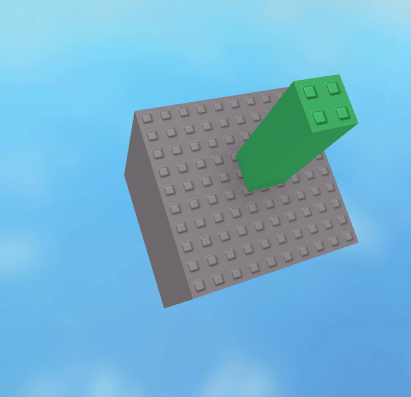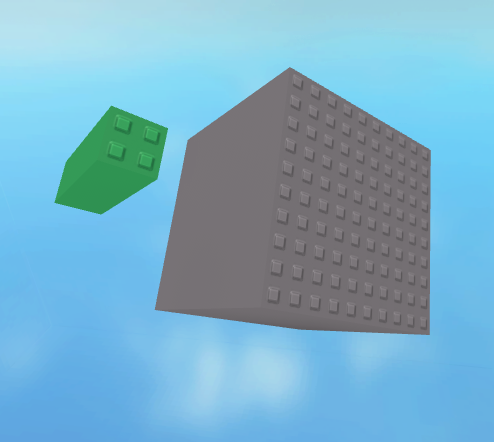# VectorToObjectSpace for relative movements like CFrame.UpVector

I want to use VectorToObjectSpace for relative directional movements.
I thought a Unit Vector of (0,1,0) ToObjectSpace would be equal to CFrame.UpVector.

``````local direction = Vector3.new(0,1,0)
local directionRelative = workspace.Origin.CFrame:VectorToObjectSpace(direction)
workspace.target.CFrame = workspace.Origin.CFrame + directionRelative*10
``````seems to work just like UpVector at first for basic rotationsbut rotate Y axis and offsets start to occur

So what do I misunderstand

Well, it’s because when you rotate on any axis that may shift the “Top” face of the Part, you’re changing that parts UpVector.

Instead of using static directions, the proper way to do this is through “Distance”

And also to note, when you use CFrame:ToObjectSpace(), you have to pass in a CFrame not a Vector3.

Finally, the alternative (for Vector3s) CFrame:VectorToObjectSpace() doesn’t provide rotation so it’ll offset, but it’ll be stuck in static world direction.
So instead we just simply use the literal UpVector as our offset. After all, it is a unit vector.

Try this:

``````local direction = workspace.Origin.CFrame.UpVector
workspace.target.CFrame = workspace.Origin.CFrame + direction*10
``````

Do let me know if I got anything a bit mixed up.
Wasn’t entirely sure what you meant by relative directional movements.

I was using VectorToObjectSpace I just mistyped the example code.
I need more than CFrame.UpVector. I was using that as a simple example and I’m still not sure why Vector3(0,1,0) in ObjectSpace is not equal to CFrame.UpVector. I have a direction that I want made relative to the CFrame of an origin part, then make a new CFrame from the origin and add the direction to it. I can find ways to do it with CFrame.Right/Up/LookVector but if VectorToObjectSpace worked the way I thought it would greatly simplify the code.

You want to use VectorToWorldSpace here

The way you mean to use it, that (0,1,0) vector is already in object space—it’s “up”, from the perspective of the object.

``````cf.UpVector == cf:VectorToWorldSpace(Vector3.new(0,1,0))
Vector3.new(0,1,0) == cf:VectorToObjectSpace(cf.UpVector)
``````
2 Likes